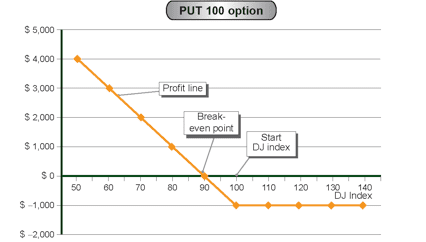# Strategy Number 3 – Long Put Option

 Market dATA DJ Index 100 points Prices Put 100 \$1,000

Strategy name:

Long Put option

Recommended use of strategy

Expectation of decline in DJ Index

Strategy components

Example: Long Put 100 at a price of \$1,000

Expenses / Income from building the strategy (at start date)

Expenditure of \$1,000

Strategy graph:Auxiliary table for building the profit line

 DJ Index (Horizontal axis) (Fixed expense) / fixed income Variable expenses Variable income (Put contribution) Total profit / (loss) (Vertical axis) 2+3+4      50 (\$1,000) – \$5,000 \$4,000 60 (\$1,000) – \$4,000 \$3,000 70 (\$1,000) – \$3,000 \$2,000 80 (\$1,000) – \$2,000 \$1,000 90 (\$1,000) – \$1,000 \$0 100 (\$1,000) – – (\$1,000) 110 (\$1,000) – – (\$1,000) 120 (\$1,000) – – (\$1,000) 130 (\$1,000) – – (\$1,000) 140 (\$1,000) – – (\$1,000)

Source of profit

The profit arises from the Put option, when the DJ Index declines below the strike index (100 points). The profit increases as the index declines.

Source of loss

Cost of building the strategy – \$1,000

Break-even point

The point where the losses from the Put option equal the cost of buying it at a price of \$1,000. This occurs when the index is at 90 points.